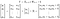# Bayesian Linear Regression with SGD, Adam and NUTS in PyTorch

PyTorch has gained great popularity among industrial and scientific projects, and it provides a backend for many other packages or modules. It is also accompanied with very good documentation, tutorials, and conferences. This blog attempts to use PyTorch to fit a simple linear regression via three optimisation algorithms:

• Stochastic Gradient Descent (SGD)
• Adam
• No-U-Turn Sampler (NUTS)

We will start with some simulated data, given certain parameters, such as weights, bias and sigma. The linear regression can be expressed by the following equation:Matrix notations of a linear regression

where the observed dependent variable Y is a linear combination of data (X) times weights (W), and add the bias (b). This is essentially the same as the nn.Linear class in PyTorch.

1. simulate data

We need to load the dependent modules, such as torch, jax, and numpyro.

`from __future__ import print_functionimport torchimport torch.nn as nnfrom torch.autograd import Variablefrom torch.distributions.normal import Normalfrom torch.distributions.uniform import Uniformfrom torch.distributions.exponential import Exponentialimport jaxfrom jax import randomfrom jax import grad, jitimport jax.numpy as np # using jax.numpy insteadimport numpyroimport numpyro.distributions as distfrom numpyro.infer import MCMC, NUTS`

Let’s simulate the data with pre-defined parameters. Here we have 50 observations, 2 weights, 1 bias, and 1 sigma.

`N = 50; J = 2X = random.normal(random.PRNGKey(seed = 123), (N, J))weight = np.array([1.5, -2.8])error = random.normal(random.PRNGKey(234), (N, )) # standard Normalb = 10.5y_obs = X @ weight + b + errory = y_obs.reshape((N, 1))X = jax.device_get(X) # convert jax array into numpy arrayy = jax.device_get(y) # convert jax array into numpy arrayx_data = Variable(torch.from_numpy(X), requires_grad=True)y_data = Variable(torch.from_numpy(y), requires_grad=True)`

2. Models

(1) SGD

The first optimisation method is SDG, and it updates the parameters in the directions of gradients of each parameters so as to minimise the loss function, such as nn.MSELoss in this case. The chunk below defines your own class of linear model.

`class lm_model(nn.Module):    def __init__(self, in_features, out_features, bias=True):        super().__init__()        self.in_features = in_features        self.out_features = out_features        self.bias = bias        self.weight = torch.nn.Parameter(torch.randn(out_features, in_features))        self.bias = torch.nn.Parameter(torch.randn(out_features))    def forward(self, input):        x, y = input.shape        if y != self.in_features:            print(f'Wrong Input Features. Pls use tensor with {self.in_features} Input Features')            return 0        output = input.matmul(self.weight.t())        if self.bias is not None:            output += self.bias        return output`

We can run the analysis:

`model = lm_model()criterion = nn.MSELoss(reduction="sum")optimizer = torch.optim.SGD(model.parameters(), lr = 0.01)for epoch in range(8000):    y_predict = model(x_data)     loss = criterion(y_predict, y_data)    if (epoch + 1) % 2000 == 0 or epoch % 2000 == 0:        print(epoch)        print("Estimated weights: ", model.linear.weight.data)        print("Estimated bias: ", model.linear.bias.data.item())        print("Estimated loss: ", loss.data.item())        print("====================")    optimizer.zero_grad()    loss.backward()    optimizer.step()`

(2) Adam

We can also define your model with a likelihood-based method, and search for the parameters to maximise the likelihood function via Adam. In other words, with the data observed (x and y), we are looking for a set of parameters, alpha, beta and sigma, to maximise the likelihood function of a Normal distribution.Likelihood-based method for a linear regression
`class lm_model_lik(nn.Module):    def __init__(self):        super().__init__()        ws = Uniform(-10, 10).sample((3, 1))        self.weights = torch.nn.Parameter(ws)        self.sigma = torch.nn.Parameter(Uniform(0, 2).sample())        def forward(self, input, output):        prior_weights = Normal(0, 10).log_prob(self.weights).sum()        prior_sigma = Exponential(2.0).log_prob(self.sigma)        i, _= input.shape        y_hat = input @ self.weights[0:2] + self.weights[2:3]        y_hat = y_hat.view(i, -1)        lik = Normal(y_hat, self.sigma).log_prob(output).sum()        LL = lik + prior_weights + prior_sigma        return -LL`

We can run the analysis with the Adam sampler:

`model = lm_model_lik()optimizer = torch.optim.Adam(model.parameters(), lr=0.01)for epoch in range(10000):    neg_log_lik = model(x_data, y_data)    if (epoch + 1) % 1000 == 0 or epoch % 1000 == 0:        print(epoch)        print("Estimated beta1: ", model.weights.data.view(3))        print("Estimated sigma: ", model.sigma.item())        print("Estimated neg logLik: ", neg_log_lik.item())        print("====================")    optimizer.zero_grad()    neg_log_lik.backward()    optimizer.step()`

(3) NUTS

It is also possible to use the NUTS to estimate the parameters in the linear model. This can be either likelihood-based (see below) or distance-based with a loss function (akin to Approximate Bayesian Computation).

`def model(X, y=None):    ndims = np.shape(X)[-1]    ws = numpyro.sample('betas', dist.Normal(0.0,10*np.ones(ndims)))    b = numpyro.sample('b', dist.Normal(0.0, 10.0))    sigma = numpyro.sample('sigma', dist.Uniform(0.0, 10.0))    mu = X @ ws + b    return numpyro.sample("y", dist.Normal(mu, sigma), obs = y)`

The model is fitted by the NUTS sampler.

`X = jax.device_put(X) # convert numpy array into jax arrayy = jax.device_put(y) # convert numpy array into jax arraynuts_kernel = NUTS(model)num_warmup, num_samples = 500, 500mcmc = MCMC(nuts_kernel, num_warmup, num_samples)mcmc.run(random.PRNGKey(0), X, y = y_obs)mcmc.get_samples()`

Useful links:

Programming, Data analysis & Deep learning!

Programming, Data analysis & Deep learning!